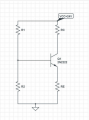# Amplifier design using BJT

#### nahavt

Joined Jun 9, 2020
1For the circuit above $$Vc=16V,β=200,VT=25mV \quad and \quad | VBE|=0.6V$$
are given. I want to design a circuit with following conditions

1. The current on R1R1 will be 0.1*Ic1. Pmax (DC maximum power consumption) =10mW
2. there will be no resistance value higher than 600kΩ
I tried to solve it using KVL and KCL but each time I got stucked with voltage drop across BJT's itself because I could not find IBIB. Are there any suggestions for how could I find it?
P.S: I do not ask for anybody to solve whole problem. I just want suggestions to solve by myself. So please just guidance, no complete solution.

•DickCappels

#### Delta prime

Joined Nov 15, 2019
558
Try the 2n2222 Datasheet.#### MrAl

Joined Jun 17, 2014
7,849
View attachment 209232

For the circuit above $$Vc=16V,β=200,VT=25mV \quad and \quad | VBE|=0.6V$$
are given. I want to design a circuit with following conditions

1. The current on R1R1 will be 0.1*Ic1. Pmax (DC maximum power consumption) =10mW
2. there will be no resistance value higher than 600kΩ
I tried to solve it using KVL and KCL but each time I got stucked with voltage drop across BJT's itself because I could not find IBIB. Are there any suggestions for how could I find it?
P.S: I do not ask for anybody to solve whole problem. I just want suggestions to solve by myself. So please just guidance, no complete solution.

It looks to me that you dont have to use a spice model just a simpler model for the transistor where you have 0.6v Vbe drop. If you have to use a spice model then you'll have to mention that.
If you can use a current controlled current source you can model the transistor like that. The base current is the controlling current and the collector current is the controlled current.

You can start by calculating the maximum current you can draw from the power supply which is 24 volts. If you can calculate the maximum current then the current though R1 is 1/10 of that according to one of the specs. You can calculate the collector current knowing Vcc and Vc and the resistance of Rc which you can calculate knowing the max current draw from the power supply and the current through R1.

Do you know what to do with the thermal voltage?

See how far you can get now.

#### MrChips

Joined Oct 2, 2009
22,061
You are missing some design specifications.
Knowing the specified input and output resistance and signal gain would be helpful.

However, here is a starter.
With 24V supply and 10mW power limitation, what is the max current you can consume?
Knowing this you can calculate a possible value for Rc.